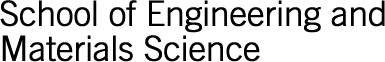Close

A note on cookiesSchool of Engineering and Materials Science Research Student Awards

PhD Thesis: Weight function formalism applied to crack bridging problems

Author: BASOALTO, Hector

Year: 1996

Supervisor(s): Ralph Stevens

The thesis is concerned with the determination of the bridging stress field that arises from the existence of bridging ligaments on the crack faces. The method presented here is based on a cuttin procedure, in which the displacements at the edge or mouth of the crack are maintained constant by reduction of the external load as the bridging ligaments are progressively removed. It provides a means of calculating the entire closure stresses due to bridging. The closure stress was assumed to be composed of a discrete distribution of tractions, so that we are forced to solve an integral equation in the displacements. The load ratio Cw(x) between the load produced by the removed traction located at x = xk over the length xk x xk + x, and the change in the external load required to keep the crack mouth displacements constant was determined. The Cw(x) was calculated using weight functions, which are proving to be a powerful tool in solving problems in fracture mechanics.
From this the bridging stress intensity factor can be determined for each of the removed tractions so that the total K can be obtained by summation of the individual K's. However, the calculations can be greatly simplified if the assumption of a straight crack profile is made, which allows (falsely) the equality of the stress intensity factor of two non-identical stress systems. In the present paper the experimental results obtained from Refs.[62,63] for the growth of fatigue cracks in alumina are analysed and discussed using weight function and straight crack formalisms. Furthermore, theoretical calculations simulating the cutting experiments, done by assuming a stress/separation function and solving the integral equation, will serve as means of comparing the approximated methods presented here with the exact results.